# Colleges with the lowest SAT scores in Washington

Top 10 colleges in Washington with the lowest SAT scores
Looking for the colleges with the lowest SAT scores in Washington? Well you're in luck! We've compiled a national college database and have created a list of the top 10 universities with the lowest SAT scores in Washington below. If you are not a good test taker or worried about your test scores, this list is for you. These are the schools whose applicants had the lowest average SAT scores in Washington, which means that you can get into these colleges with a lower SAT score. We also include each college's ACT scores and acceptance rate so that you can see where you would have the easiest time getting in. Read on to find out more.

## Eastern Washington University SAT scores

The average SAT score for Eastern Washington University is 980.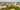The average SAT score of 980 breaks down into:

• SAT math: 480

The average ACT score for Eastern Washington University is 20 and their acceptance rate is 63.5%.

## Central Washington University SAT scores

The average SAT score for Central Washington University is 1031.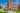The average SAT score of 1031 breaks down into:

• SAT math: 510

The average ACT score for Central Washington University is 19 and their acceptance rate is 64.4%.

## The Evergreen State College SAT scores

The average SAT score for The Evergreen State College is 1110.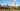The average SAT score of 1110 breaks down into:

• SAT math: 530

The average ACT score for The Evergreen State College is 24 and their acceptance rate is 95.2%.

## Washington State University SAT scores

The average SAT score for Washington State University is 1125.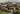The average SAT score of 1125 breaks down into:

• SAT math: 560

The average ACT score for Washington State University is 23 and their acceptance rate is 75.8%.

## Saint Martin's University SAT scores

The average SAT score for Saint Martin's University is 1130.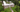The average SAT score of 1130 breaks down into:

• SAT math: 560

The average ACT score for Saint Martin's University is 21 and their acceptance rate is 95.6%.

## Walla Walla University SAT scores

The average SAT score for Walla Walla University is 1140.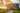The average SAT score of 1140 breaks down into:

• SAT math: 550

The average ACT score for Walla Walla University is 24 and their acceptance rate is 86%.

## University of Washington Bothell SAT scores

The average SAT score for University of Washington Bothell is 1145.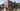The average SAT score of 1145 breaks down into:

• SAT math: 580

The average ACT score for University of Washington Bothell is 23 and their acceptance rate is 79.4%.

## Pacific Lutheran University SAT scores

The average SAT score for Pacific Lutheran University is 1170.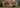The average SAT score of 1170 breaks down into:

• SAT math: 580

The average ACT score for Pacific Lutheran University is 24 and their acceptance rate is 79.8%.

## Western Washington University SAT scores

The average SAT score for Western Washington University is 1179.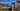The average SAT score of 1179 breaks down into:

• SAT math: 580

The average ACT score for Western Washington University is 25 and their acceptance rate is 87.7%.

## Seattle Pacific University SAT scores

The average SAT score for Seattle Pacific University is 1190.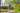The average SAT score of 1190 breaks down into:

• SAT math: 570